# The Importance of Skip Counting

## What is skip counting?

Skip counting is the method of counting forwards by a number that is not 1. TO skip count, you would add the same number each time to the previous number.

For example: skip counting by 2. Let us show you that on a number line: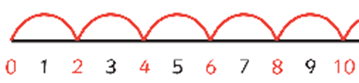## Why is skip counting important?

Essentially, skip counting helps you to:

• Count many things quickly;

However, skip counting is the foundation to learn other math concepts covered in higher grades, including:

• Multiplication
• Division
• Lowest Common Factor
• Greatest Common Factor
• Reducing Fractions
• Factoring
• Prime and Composite Numbers
• Recognizing Number Patterns and Sequences

## Skip counting worksheets

Skip counting is such an essential skill to learn that it’s covered across several grades. We have created worksheets for students in kindergarten to grade 3 to practice skip counting at increasingly harder levels.

## Kindergarten skip counting by 2s and 10s practice

For late kindergarten use, these worksheets help students practice skip counting by 2 and 10.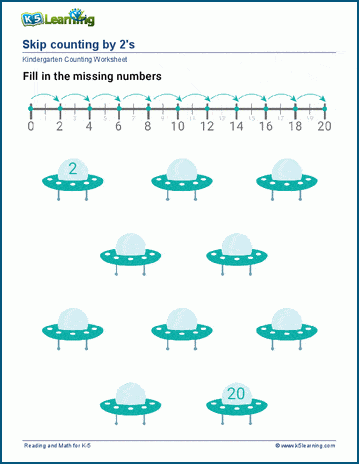## Counting by 2, 5 and 10 for grade 1 students

Students fill in the missing numbers for skip counting by 2, 5 and 10.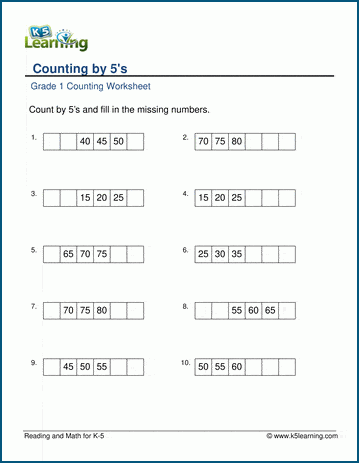## Grade 1 counting by 3, 4, 5, and 10 worksheets

Using number charts, students count by 3, 4, 5 and 10.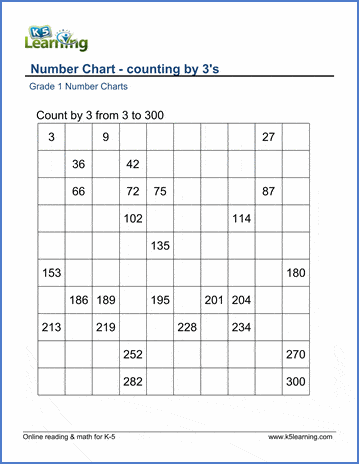## Grade 2 skip counting by 10 worksheets

We have separate worksheets for skip counting by 10 starting at 10, by 10 from 1-10, and 10 for 1-100.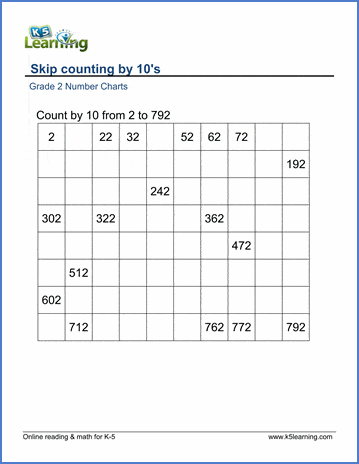## Skip count by 20, 25, 50 and 100 practice for grade 2 students

The next section of worksheets, has students practice skip counting by 20, 25, 50 and 100.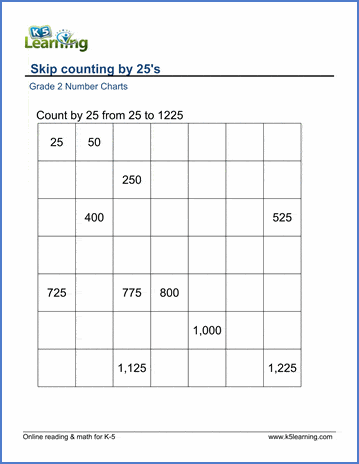## Skip count by 2, 4, 5, 6, 7, 8, and 9 for grade 2

Then, students work on skip counting by 2 (even and odd) and 3, 4, 5, 6, 7, 8 and 9.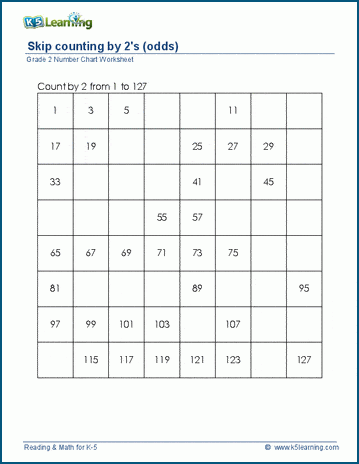## Skip count by 100 starting from random numbers practice for grade 3

In number charts, students skip count by 100, starting from a random number.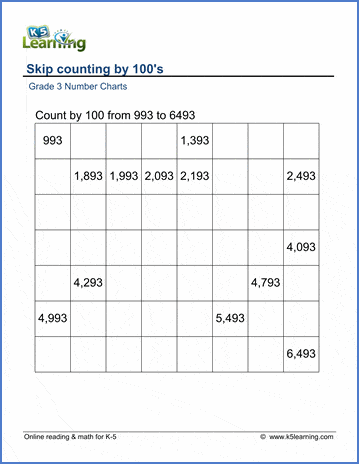## Grade 3 skip count by 150, 200 and 250 worksheets

The final section of worksheets practice skip counting by 150, 200 and 250, starting from 0.